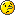# Same parameters for two different fit functions

Dear ROOTERs,

I have a problem with my fit function. I have two different fit functions for different data ranges and I would like to make some parameters the same for both functions.
I have made a small example with two different Gaussian functions. In principle I want the norm or the standard deviation to be the same after the fitting process but optimized for both data ranges at the same time. Of course, in this simple example I could use a single fit function but this is not possible in my application.

Hope someone has a solution for this problem.
two_Gaussians.c (957 Bytes)

Hi,

what you are trying to carry out is a simultaneous fit.
You can have a look to root.cern.ch/root/html/tutorial … Fit.C.html

Cheers,
Danilo

Thanks for the hint Danilo. The simultaneous fit example is very nice, I almost solved my problem with it but still wouldn’t be the perfect solution. I guess the example is more for a combined fit for two different data sets.

I hoped to hear that there is a solution like:

Double_t combined(Double_t *x, Double_t *par){
return gaus1(x,par)+gaus2(x,&par[3,4,2])
}
what should mean that the standard deviation for both is the same. However, I finally found that my function is not as complicated as expectedand solved my problem like this:

Double_t complicated_function(Double_t *x, Double_t *par){
Double_t val;
if (x<par) val=function1(x,par[i]);
else val= function2(x,par[i]);
if (x<par) return val+function3(x,par[i]);
else return val+function4(x,par[i])
}

where [i] is an arbitrary set of mixed parameters.

Thanks,
Don